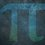# Triangles...

Area of a triangle (one even and one odd length): $\frac{b}{2}$ $\times h$ or $b \times$ $\frac{h}{2}$

Example $1$:

$b = 8$

$h = 9$

Method $1$ (Normal):

$8 \times 9 = 72 \div 2 = 36$

Method $2$ (Mine):

$\frac{8}{2}$ $\times 9 = 4 \times 9 = 36$

Example $2$:

$b = 7$

$h = 2$

Method $1$ (Normal):

$7 \times 2 = 14 \div 2 = 7$

Method $2$ (Mine):

$7 \times$ $\frac{2}{2} = 7 \times 1 = 7$Note by Yajat Shamji
2 months, 4 weeks ago

This discussion board is a place to discuss our Daily Challenges and the math and science related to those challenges. Explanations are more than just a solution — they should explain the steps and thinking strategies that you used to obtain the solution. Comments should further the discussion of math and science.

When posting on Brilliant:

• Use the emojis to react to an explanation, whether you're congratulating a job well done , or just really confused .
• Ask specific questions about the challenge or the steps in somebody's explanation. Well-posed questions can add a lot to the discussion, but posting "I don't understand!" doesn't help anyone.
• Try to contribute something new to the discussion, whether it is an extension, generalization or other idea related to the challenge.

MarkdownAppears as
*italics* or _italics_ italics
**bold** or __bold__ bold
- bulleted- list
• bulleted
• list
1. numbered2. list
1. numbered
2. list
Note: you must add a full line of space before and after lists for them to show up correctly
paragraph 1paragraph 2

paragraph 1

paragraph 2

[example link](https://brilliant.org)example link
> This is a quote
This is a quote
    # I indented these lines
# 4 spaces, and now they show
# up as a code block.

print "hello world"
# I indented these lines
# 4 spaces, and now they show
# up as a code block.

print "hello world"
MathAppears as
Remember to wrap math in $$ ... $$ or $ ... $ to ensure proper formatting.
2 \times 3 $2 \times 3$
2^{34} $2^{34}$
a_{i-1} $a_{i-1}$
\frac{2}{3} $\frac{2}{3}$
\sqrt{2} $\sqrt{2}$
\sum_{i=1}^3 $\sum_{i=1}^3$
\sin \theta $\sin \theta$
\boxed{123} $\boxed{123}$

Sort by:

Nice! Just remember when working out don't say 89 = 72/2 cause that means 89=36!

- 2 months, 3 weeks ago

Thanks!

- 2 months, 3 weeks ago

b/2 x h is the same old triangle area formula. I'm seeing a lot of these notes that are pretty redundant lately.

- 2 months, 3 weeks ago

- 2 months, 3 weeks ago

No, it isn't - it's a variation...

- 2 months, 3 weeks ago

$\text{\Huge It doesn't have to be one even and one odd; it doesn't even have to be integers.}$

- 2 months, 3 weeks ago

I am just showing it for integers to show that you can alter the formula.

- 2 months, 3 weeks ago